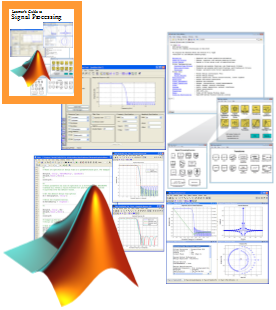Training on Signal Processing and Filter Design with MATLAB & Simulink Implementation Solutions 4U Training Center @ Puchong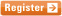11 & 12 MAR, 2021
" Comprehensive in scope and clear in approach,
this workshop keeps math at a tolerable level, and makes
signal processing exceptionally accessible
without ever oversimplifying it..."
 Signal may be referred to as a function of time that conveys information about the behavior or characteristic of some physical phenomena. Examples of signal are temperature, voice, communication and geophysical among others. Signal can be acquired from its physical environment via sensors (e.g. microphone for voice acquisition). Nevertheless, the acquired signal is less than ideal that may be resultant from constraints of the acquisition device or due to background noise pollution. Therefore, signal-processing comprises of useful techniques in analyzing the characteristics of the acquired signal and filtering unwanted components from it. Ultimately, the main task of signal-processing involves filter design, either via infinite-impulse-response (IIR) or finite-impulse-response (FIR) techniques, such as to achieve better signal quality. This course is conducted in a workshop-like manner, with a balance mix of theory, hands-on coding and simulation using MATLAB and Simulink. Extensive exercises are provided throughout the course to ensure students’ familiarity in visualizing, processing and filtering signals by using MATLAB and Simulink.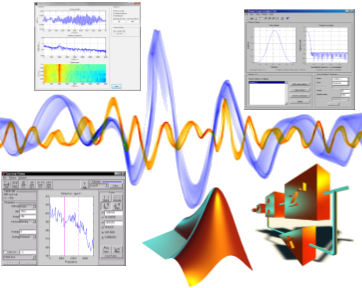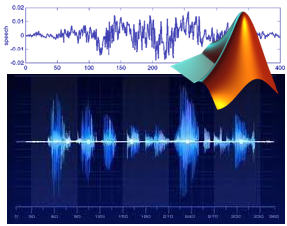Course highlights This course is intended as a practical introduction to signal-processing concepts and its implementation using MATLAB and Simulink. As such, there will be a series of hands-on exercises which support both theoretical explanation and practical implementation. What you will learn Represent signals via data import, simulation and the conversion to digital signals via sampling. Visualization of signals in time- as well as in frequency-domains via discrete Fourier transformation. Understand the concept of linear-time-invariant (LTI) system and its representation in z-domain and its visualization via pole-zero plot. Understand the concept of infinite-impulse-response (IIR) and finite-impulse-response (FIR) and familiar with the process of IIR and FIR filter design.

Course methodology

The course begins with guidance on how to represent signals in both analog and digital forms, visualization of signals in both time- and frequency-domains, understanding of LTI concepts as well as designing filters using MATLAB and Simulink. The course will be well-supported by MATLAB codes and Simulink models.

Course Outline

Day 1

• Introduction
• Concept of signals
• Analog signals
• Discrete signals
• Sampling of signals
• Types of discrete signals
• Noise modeling
• Spectral analysis
• Frequency domain
• Fourier transform (DFT and FFT)
• Power spectral density
• SPTool for signal analysis
• Signal import and visualization
• Spectral analysis
• Concept of Linear-Time-Invariance (LTI)
• Representation of LTI system – difference equation
• Representation of LTI system – block diagram
• The z-Transform
• Representation via transfer function
• Pole-zero plots
• Case studies and exercises

Day 2

• Analog prototyping
• Butterworth
• Chebyshev
• Elliptic
• Filter transformation
• Impulse-invariant method
• Bilinear transformation method
• Case study - design of IIR filter:
• Based on filter specifications
• Based on signal characteristics
• Introduction to windowing technique
• Types of windows
• Characteristics of windows
• Procedures of FIR filter design
• Case study – design of FIR filter:
• Based on filter specifications
• Based on signal characteristics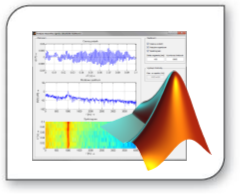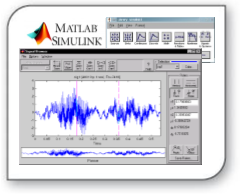Who should attend

Professional, engineers and scientists at all levels who wish to learn about concepts of signal-processing and its implementation using MATLAB and Simulink. No background in either of these topics are, however, assumed. The detailed course material and many source code listings will be invaluable for both learning and reference.

Prerequisites

A basic familiarity with MATLAB and Simulink environment is preferred.

Note: Due to the nature of the course and the learning expectations, the availability seats are limited. You need to register early to obtain confirmation of your space.